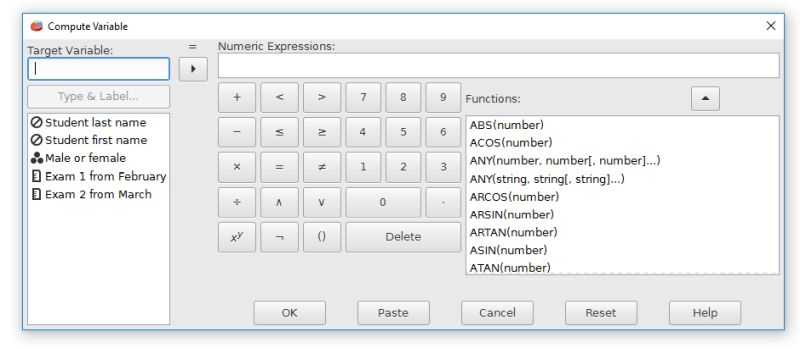# PSPP for Beginners

## Compute Variable

Sometimes it is useful to create a new variable by performing mathematical operations on existing variables. For our current example let's make a new final grade variable by adding the Exam 1 and Exam 2 variables.

The Transform menu has a Compute command. The dialog box for this Compute Variable command looks like this:The Target Variable field needs a name for a new variable. Let's call it FinalGrade.

The Numeric Expression field is the mathematical operation. You can use variables with mathematical operators. For our example we will add Exam1 and Exam2. The variables can be moved to the numeric expression field by using the arrow key (outlined in blue). Note that using advanced mathematical operations is possible by choosing these analyses from the functions list.Clicking the "OK" button will create a new variable called FinalGrade that is the sum of Exam1 and Exam2.